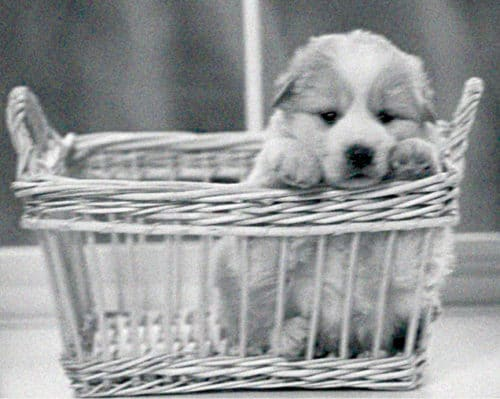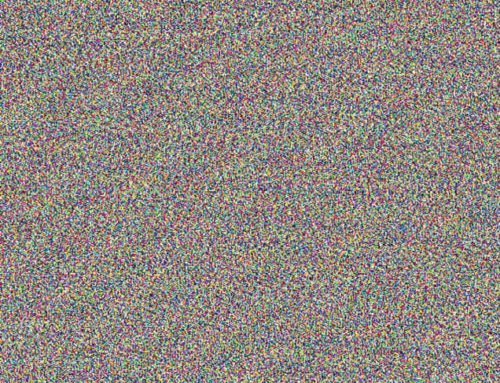# Image Processing Using MATLAB: Image Deblurring and Hough Transform (Part 4 of 4)

Dr Anil Kumar Maini is former director, Laser Science and Technology Centre, a premier laser and optoelectronics R&D laboratory of DRDO of Ministry of Defence--Varsha Agrawal is a senior scientist with Laser Science and Technology Centre (LASTEC), a premier R&D lab of DRDO

18876

## Read Part 3

Image pre-processing and identification of certain shaped objects in the image is explained here using Image Deblurring and Hough Transform.

Image deblurring removes distortion from a blurry image using knowledge of the point spread function (PSF). Image deblurring algorithms in Image Processing Toolbox include Wiener, and regularised filter deconvolution, blind, Lucy-Richardson, as well as conversions between point spread and optical transfer functions. These functions help correct blurring caused by out-of-focus optics, camera or subject movement during image capture, atmospheric conditions, short exposure time and other factors.

deconvwnr function deblurs the image using Wiener filter, while deconvreg function deblurs with a regularised filter. deconvlucy function implements an accelerated, damped Lucy-Richardson algorithm and deconvblind function implements the blind deconvolution algorithm, which performs deblurring without the knowledge of PSF. We discuss here how to deblur an image using Wiener and regularised filters.

## Deblurring using Wiener filter

Wiener deconvolution can be used effectively when frequency characteristics of the image and additive noise are known to some extent. In the absence of noise, Wiener filter reduces to an ideal inverse filter.

deconvwnr function deconvolves image I using Wiener filter algorithm, returning deblurred image J as follows:

J = deconvwnr(I,PSF,NSR)

where image I can be an N-dimensional array, PSF the point-spread function with which image I was convolved, and NSR the noise-to-signal power ratio of the additive noise. NSR can be a scalar or a spectral-domain array of the same size as image I. NSR=0 is equivalent to creating an ideal inverse filter.

Image I can be of class uint8, uint16, int16, single or double. Other inputs have to be of class double. Image J has the same class as image I.

The following steps are taken to read ‘Image_2.tif’, blur it, add noise to it and then restore the image using Wiener filter.

1. The syntax to read image (Image_2.tif) into the MATLAB workspace and display it is:
>> I=im2double(imread(‘Image_2.tif’));
>>imshow(I)Fig. 1: Image read into the MATLAB workspace

Fig. 1 shows the image generated by imshow function.

2. h=fspecial(‘motion’, len, theta) returns a filter to approximate the linear motion of a camera by len pixels, with an angle of theta degrees in a counter-clockwise direction. The filter becomes a vector for horizontal and vertical motions. The default value of len is 9 and that of theta is 0, which corresponds to a horizontal motion of nine pixels. B=imfilter(A,h) filters multidimensional array A with multidimensional filter h. Array A can be logical or non-sparse numeric array of any class and dimension. Result B has the same size and class as A. The syntax is:
>> LEN=21;
>> THETA=11;
>> PSF=fspecial(‘motion’,LEN,THETA);
>> blurred=imfilter(I,PSF,’conv’,’circular’);
>>figure,imshow(blurred)Fig. 2: Image after filtering with multidimensional filter

Fig. 2 shows the image generated by imshow function.

3. J=imnoise(I,’gaussian’,M,V) adds Gaussian white noise of mean M and variance V to image I:
>>noise_mean=0;
>>noise_var=0.002;
>>blurred_noise=imnoise(blurred,’gaussian’,
noise_mean,noise_var);
>>figure, imshow(blurred_noise)Fig. 3: Image generated after addition of Gaussian white noise

Fig. 3 shows the image generated by imshow function.

4. As mentioned above, J=deconvwnr (I,PSF,NSR) deconvolves image I using Wiener filter algorithm, returning deblurred image J. The following commands generate a deblurred image using NSR of zero:
>>estimated_nsr=0;
>> wnr2=deconvwnr(blurred_noise,PSF,
estimated_nsr);
>>figure,imshow(wnr2)Fig. 4: Deblurred image using NSR of zero

Fig. 4 shows the image generated by imshow function.

5. The following commands generate a deblurred image using NSR calculated from the image:
>>estimated_nsr=noise_var/var(I(:));
>> wnr3=deconvwnr(blurred_noise,PSF,
estimated_nsr);
>>figure,imshow(wnr3)Fig.5: Restored image from the blurred and noisy image using estimated NSR generated from the image

Fig. 5 shows the restored image from the blurred and noisy image using estimated NSR generated from the image.

Deblurring with a regularised filter

A regularised filter can be used effectively when limited information is known about the additive noise.

J=deconvreg(I, PSF) deconvolves image I using regularised filter algorithm and returns deblurred image J. The assumption is that image I was created by convolving a true image with a point-spread function and possibly by adding noise. The algorithm is a constrained optimum in the sense of least square error between the estimated and true images under requirement of preserving image smoothness.

Image I can be an N-dimensional array.
Variations of deconvreg function are given below:

• J = deconvreg(I, PSF, NOISEPOWER)
where NOISEPOWER is the additive noise power. The default value is 0.

• J = deconvreg(I, PSF, NOISEPOWER, LRANGE)
where LRANGE is a vector specifying range where the search for the optimal solution is performed.

The algorithm finds an optimal Lagrange multiplier LAGRA within LRANGE range. If LRANGE is a scalar, the algorithm assumes that LAGRA is given and equal to LRANGE; the NP value is then ignored. The default range is between [1e-9 and 1e9].

• J = deconvreg(I, PSF, NOISEPOWER, LRANGE, REGOP)

where REGOP is the regularisation operator to constrain deconvolution. The default regularisation operator is Laplacian operator, to retain the image smoothness. REGOP array dimensions must not exceed image dimensions; any non-singleton dimensions must correspond to the non-singleton dimensions of PSF.

[J, LAGRA] = deconvreg(I, PSF,…) outputs the value of Lagrange multiplier LAGRA in addition to the restored image J.

In a nutshell, there are optional arguments supported by deconvreg function. Using these arguments you can specify the noise power value, the range over which deconvreg should iterate as it converges on the optimal solution, and the regularisation operator to constrain the deconvolution.